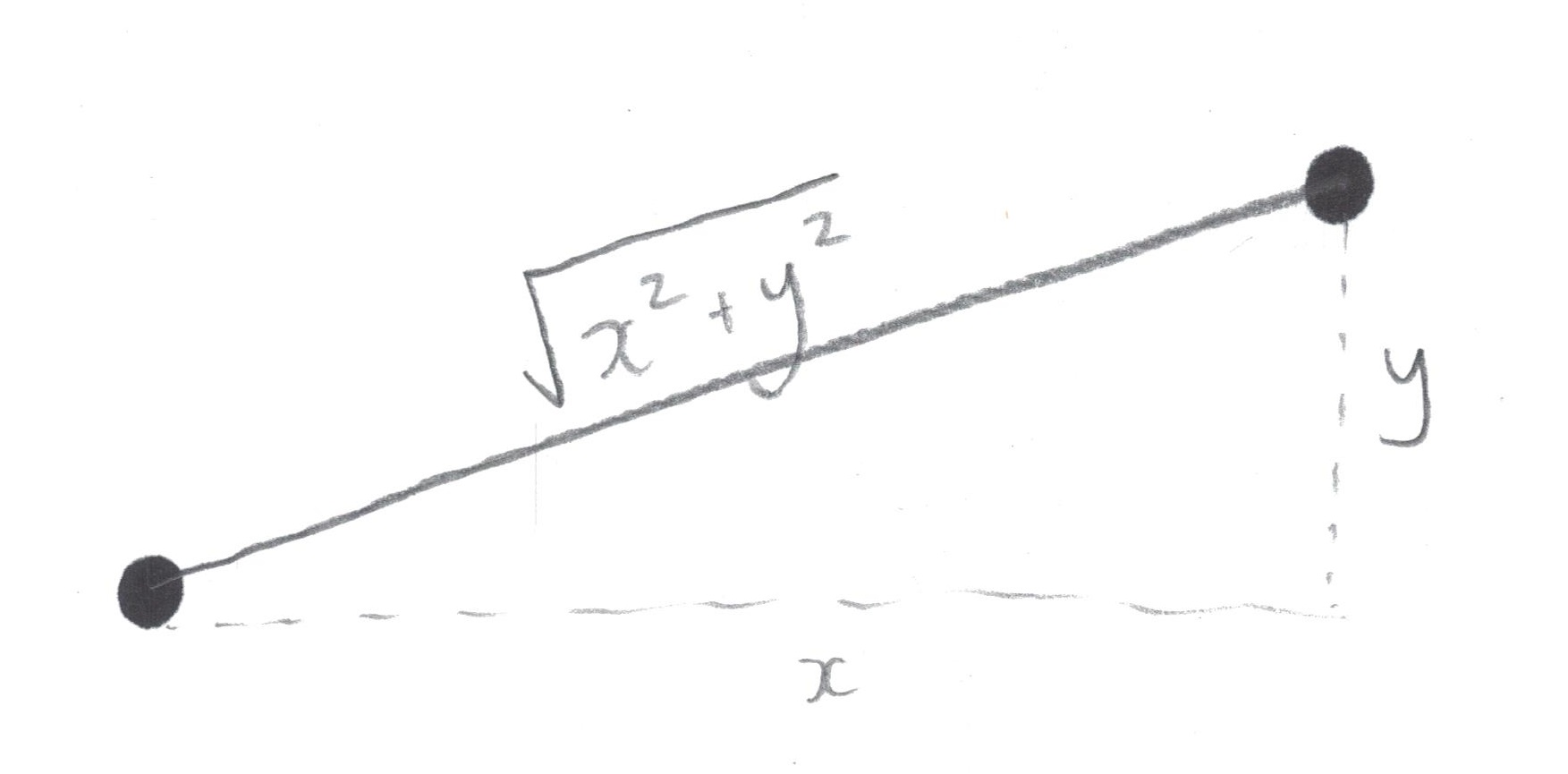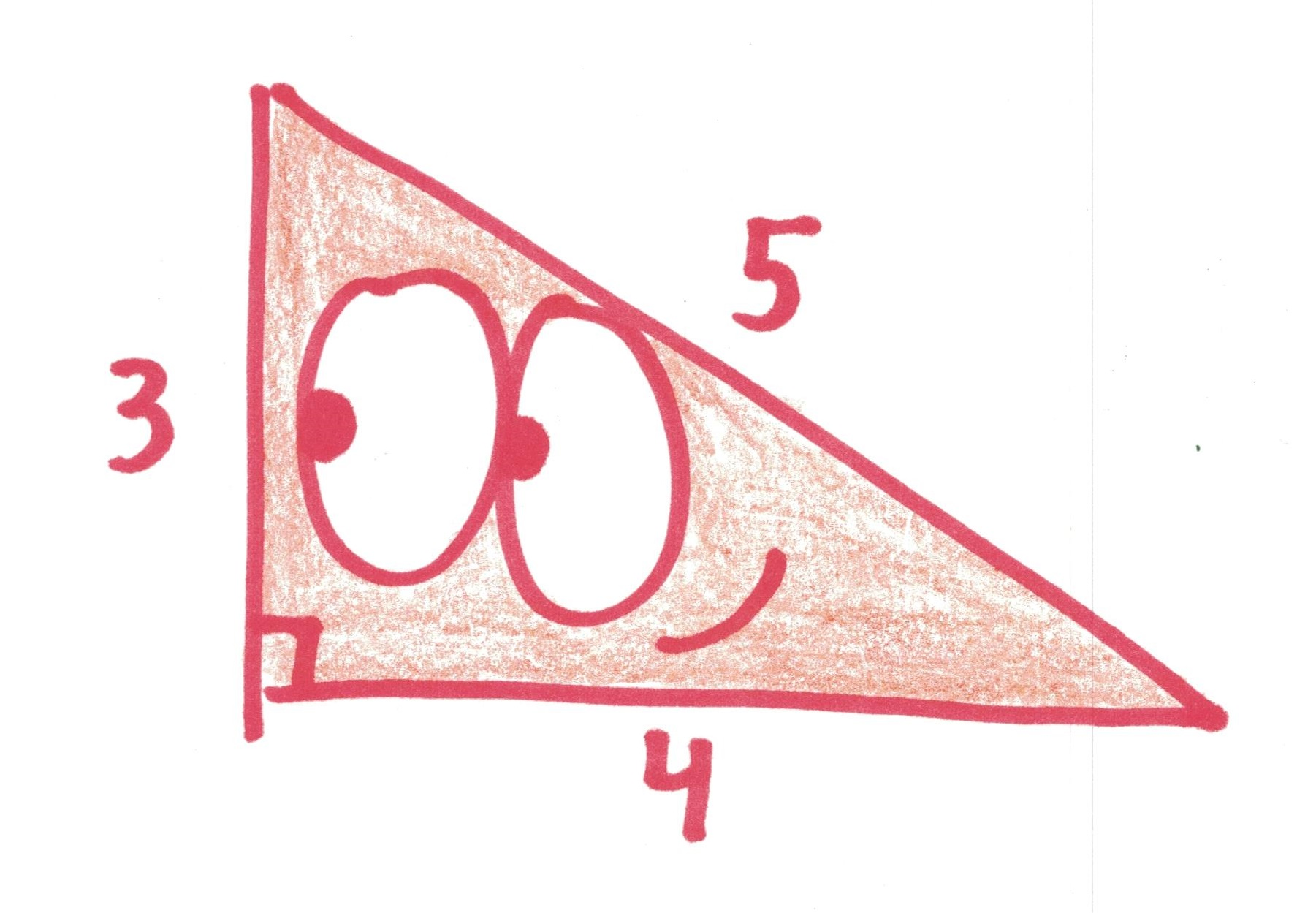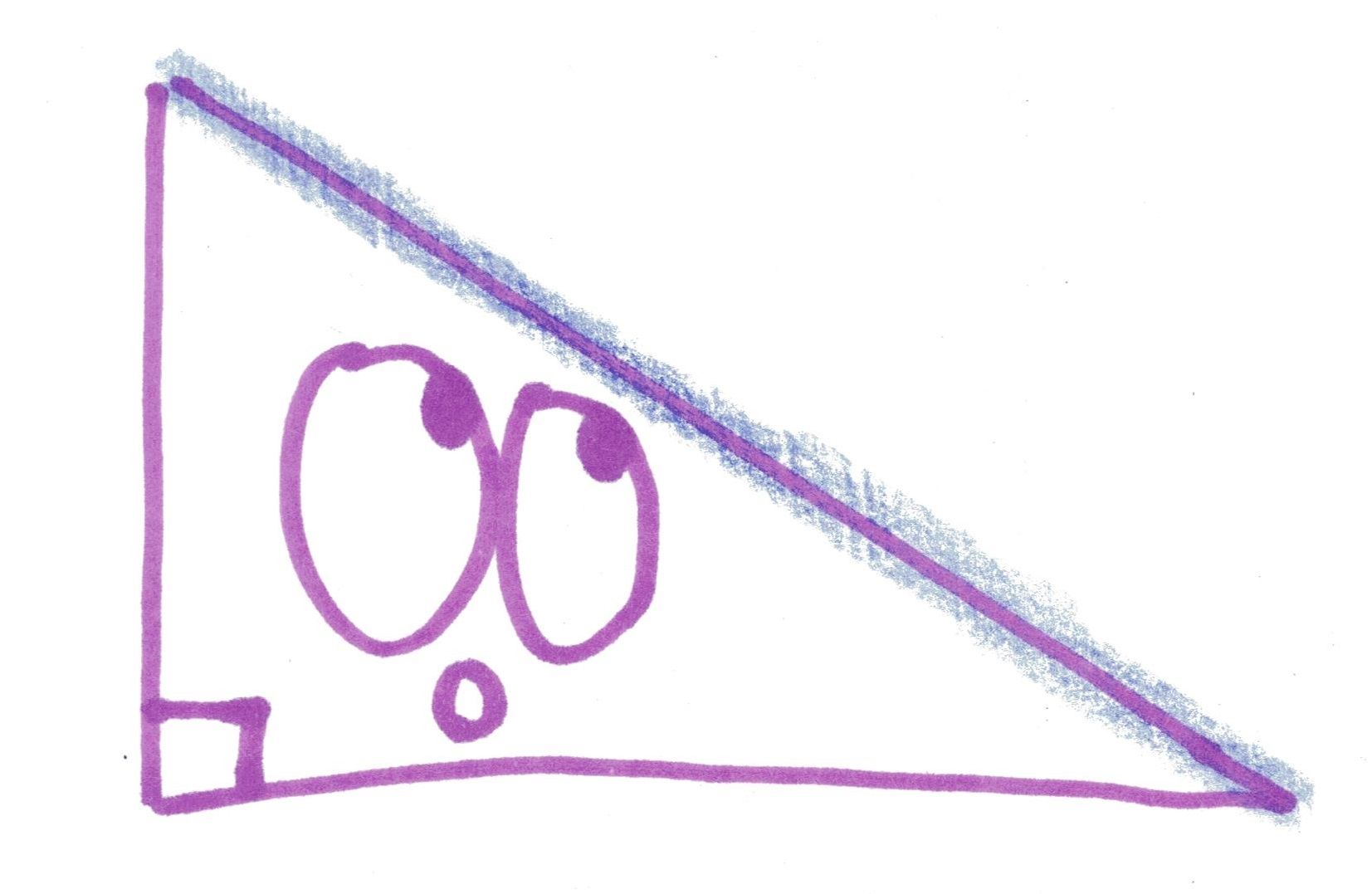# Let’s Rename the Pythagorean Theorem

Pythagoras was many wonderful things. A delirious mystic. A benevolent cult leader. A bean-hating vegetarian. A real person (maybe).

One thing he was not: the guy who gave us the Pythagorean Theorem.

So why does he get his name on it? I cry foul. I cry “no more.” I cry, “Let us band together and vote on a better name for this ancient theorem! Not because it will actually result in a name change, but because it’s a fun debate!”

Who’s with me?!

I submit for your consideration the following names, from Hambrecht and the other clever folks in his thread:

1. The Three Squares Theorem. Although we perceive it as a claim about numbers, for most mathematical cultures, this was a claim about shapes. To wit: if you affix squares to the sides of a right triangle, the two smaller areas add up to equal the largest.2. The Babylonian Formula. Give credit where credit is due! As Hambrecht says, this name “has the hint of far-away times and places… Through millenia and continents, this piece of math connects us to strange, alien people, yet so much our equals.” He calls it “fuel for children’s imaginations.” Even more important, as an astute observer points out: it can be abbreviated as “Baby Formula.”3. The Distance Theorem. The theorem’s most ubiquitous use is in finding distances, especially in higher dimensions.4. The Huey Lewis Theorem. Proposed (or, pun-posed) by Susan Burns, because, and I quote: “It’s hyp to be square…or is that b-squared?”5. The Adrakhonic Theorem, because that’s what it’s called in Neal Stephenson’s novel Anathem (which I just added to my reading list).6. Squaring the Triangle. Olaf Doschke’s suggestion, with a ring of the famously impossible “squaring the circle.”7. The Sum of Squares Theorem. Descriptive, clear.8. Garfield’s Theorem. Because if we’re just naming it after a random dude who supplied a proof, why not pick an assassinated U.S. president?9. Theorem 3-4-5. After the most famous Pythagorean triple.10. Euclid, Book I, Proposition 47. “Like chapter and verse in the mathematical bible,” explains George Jelliss.11. The Hypotenuse Theorem. Because it’s all about that longest side.12. The Right Theorem. Because it’s all about that right angle. (Also, because it’s right.)13. The Distance/Area Theorem. Because it’s all about multiple things at once.14. The Benjamin Watson Theorem. Because of this heroic, historic tackle, brought to my attention by Fawn Nguyen in her appearance on My Favorite Theorem.

Now, we could leave it there. We could say, “This has been a fruitful discussion. Let’s call it a day!” We could say, “Obviously a random blog post isn’t going to succeed in renaming the most famous theorem in mathematics, so let’s go home and eat raisins and watch sitcom reruns like the human mediocrities we are.”

But I say no! I say it’s time for a referendum!

What say you, good people of the internet? What is the best name for this fundamental theorem of geometry?

Other ideas are, of course, welcome in the comments below.

## 46 thoughts on “Let’s Rename the Pythagorean Theorem”

1.gasstationwithoutpumps says:

What about “the square hole theorem” after my favorite proof (which has a square with side length c with rotationally symmetric triangles inside, leaving a square hole in the middle with side length b-a).

2.James Orlin says:

One name that should not be used is “The scarecrow’s theorem.” In the movie version of the Wizard of Oz, the scarecrow gets it extremely wrong. He says. “The sum of the square roots of any two sides of an isosceles triangle is equal to the square root of the remaining side.” Perhaps he was considered to have a brain because he could say something technical (albeit incorrect) with complete confidence.

3.Jim Propp says:

We could call it The Theorem of A Thousand Proofs. (Sure, only a few hundred are currently known, but I’m an optimist.)

4.Omar says:

I taught it to my daughter as the ‘right triangle formula’. It removes a step in remembering what it is for.

5.asukalin says:

People called it “Hook-Thigh Theorem(勾股定理)” in China, for “hook(勾)” is the name of the shorter leg of an right triangle while “thigh(股)” is the name of the longer one in ancient Chinese terminology system. It’s kind of a boring name, but also a simple and straightforward one.

6.mr sock monkey says:

relating to suggestment # 12 in post, monkey think “not wrong theorem” = good name.

7.Shecky R says:

Well, it’s a free country so all the riff-raff can vote, but Fawn should have the final say since it’s HER favorite theorem!

1.Paula Beardell Krieg says:

The Fawn Nguyen theorem.

8.jensilvermath says:

It’s known in the East as the Shang Gao Theorem (商高定理), circa 1046-256 BCE. see http://bit.ly/ShangGaoThm

9.cma561 says:
10.Rogers George says:

When I taught math a long time ago, we called it “Joe’s theorem” because his full name was “Joe Pythagoras.” And I got _that_ from my high school math teacher.

11.mathproblemsolvingskills says:

Where’s the Watson Theorem? That’s what I would vote for.

12.gregsdennis says:

I like Sum of Squares because it has a nice polarity with Difference of Squares (factoring polynomials).

13.Russell Helmstedter says:

You didn’t include “The Benjamin Watson Theorem” in the vote?! I’m done with you. I’m sending back the copy of your book my dog chewed up.

14.Edward Welbourne says:

So the nice thing about it as “The sum of squares theorem” is that it lets us do arithmetic with squares, adding up arbitrarily many of them and always getting a square. It is, of course, equally a difference of squares theorem: the square on the hypotenuse, minus one of the squares on the other sides, is the square on the remaining side; and this can be used to turn it into a way of taking the square root of any rectangle’s area. (So perhaps better to call it “the square arithmetic theorem”.) There are at least two other ways to turn a rectangle into a square of equal area (one using Euclid’s proof of the sum of squares theorem, one using the intersecting chords theorem) . We can cut up any polygon into triangles; and can turn any triangle into a rectangle of equal area. (Connect the mid-points of two edges; the line that does so is parallel to the third; cut along this line and recombine the pieces to make a parallelogram; shear this to a rectangle.) So we can construct squares equal in area to each of the triangles into which we cut up our polygon; and we can then add up those squares using the sum of squares theorem, to get a square equal to the polygon. Thus we can square any polygon (albeit not a circle).

I note that Garfield’s proof is simply one half of the standard diagram for a standard proof, as seen on an ancient clay tablet with the Sanskrit word for “Aha!” written under it: half-turn Garfield’s diagram about its diagonal of the square on the hypotenuse to get the square on the sum of the other two sides, with four copies of the triangle cut out from corners to leave the square on the hypotenuse; quarter-turn two of the cut out triangles to put their hypotenuses onto those of the other two, leaving the sum of the squares on the other two sides.

While we’re on the topic: has anyone here met (elsewhere) a proof by tessellation  that I came up with for myself and begin to suspect might even be original ? Make two copies of the right triangle, rotated from one another by a quarter turn about their right corner; use their respective copies of the two non-hypotenuse sides to make squares. (You have two perpendicular copies of a side; the other two sides of the square are implied.) Translate this combination of two squares through the displacements along our two copies of the hypotenuse; if you draw the picture, it’s immediately clear that the translated combinations abut and nestle with the original and each other; we can repeat these translations indefinitely to tessellate the plane. Since the same translations also tessellate the square on a hypotenuse, the unit figures the two tessellation are translating must in fact have the same area; if only by cutting a cell of one tessellation out of the other and rearranging its pieces to make the cell of that other.

15.jewelsofsayuri says:

I would simple call it the triangle theorem, as that is what I remember calling it as a kid when I first learnt it. From your choices I would pick The Hypotenuse Theorem.

1.Edward Welbourne says:

Well, the first impressions of a kid are a compelling (pedagogic) argument: how about “The right triangle theorem” though ? (Or the half-rectangle theorem ?) Sneaking a little of the teacher’s lesson into the child’s intuition may be the key to carrying more teaching into the name.

16.Luke says:

If one were forced to pick one of these, I think the best option would be the distance theorem.

I’ve always had trouble calling Pythagoras’ theorem a “theorem” though, because it isn’t a theorem—it’s the definition of distance/length. It defines the norm √(x² + y²) for ℝ², but one could just as easily take ∛(x³ + y³) instead, or even max{|x|,|y|}.

Just because the usual norm/metric happens to be “God’s favourite metric”, in that it models reality, doesn’t mean it should be named a “theorem”, as if it’s a mathematical truth.

1.Edward Welbourne says:

The original geometric statement (about areas of squares constructed around a triangle) is definitely a theorem, not a definition. Things can have lengths a priori to imposing co-ordinates on the space in which you find them (and then expressing the lengths in terms of those co-ordinates, exploiting this theorem to show the expression is coherent); we can compare, bisect and repeat angles a priori to describing them in terms of a metric on the co-ordinates of lines. The assorted norms, on a space of lists of co-ordinates, that satisfy the triangle inequality, are interesting (and sporadically useful) notions of distance that satisfy the needs of topologists, but geometry (rather than God) happens to fit nicely with the L2 norm, precisely because of this sum of squares theorem. Indeed, this is one of the reasons why the co-ordinate representation of Euclidean space is so effective and practical. You wouldn’t have been looking at ℝ² in the first place, but for this theorem !

1.drmenguin says:

I don’t see how you can do geometry mathematics without a notion of distance, which in turn requires a notion of position. In other words, you need both a set of points and a metric to be able to do geometry. Depends what you mean by “geometry” of course, I am assuming we are talking about the modern understanding of geometry, and not Euclid.

I don’t know of any geometric reason why the L2 norm is “special”, again, it is only of particular concern to us because it reflects the real world. You can equally do geometry valid geometry with any Lp norm for p at least 1, or with other norms.

It is purely because of this definition of distance that Euclidean geometry is the way that it is, so I would argue that this definition is the starting point, and then geometry follows.

17.Keith McClary says:

There is an important theorem that $\lim_{n \to \infty}(1 +\frac{1}{n})^n \lt \infty$ . The limit has a name: $e$, but the theorem has no name.

18.itsmayurremember says:

I vote The Right Theorem

19.jburne says:

I can’t believe I’m the first to suggest “Law of Cosines, Special case.”

20.Dylan says:

We could call it the “Fundamental Theorem of Geometry” as you joked about it being at the end of your article. 😛 Of the options presented, I like the three squares theorem the most. (Although the theorem still holds true if you use any three similar shapes instead of squares.)

21.Steve Spivey says:

From the video, it appears that the ball is caught at about 2 yards into the end zone. The other player was at the 8 yard line at around 44.5 yards across the field.

He had to run ~101.3 yards in the same time the carrier ran 102, IF he went completely straight.

22.Will Orrick says:

Jens Høyrup, who is a historian of ancient mathematics, calls it the “diagonal rule”. In the Old Babylonian period, the rule seems to have been understood as the method for finding the length of the diagonal of a rectangle in terms of the lengths of the sides, hence the name. This also appears to be how the rule was formulated in the Shulba Sutras, that is, in terms of rectangles rather than right triangles.

23.estelle says:

I like number 9 its simple and easy to remember and also includes all sides of the shape.

24.Shawn Mansoor says:

I like theorem 3-4-5. It’s easy to remember.

25.lita says:

the triangle theorem

26.mckayla childe says:

the Babylonian Formula

27.Bengie says:

i like the babylonian formula

28.valentina says:

I like theorem 4

29.William says:

The Distance/Area Theorem is my favorite due to the fact that it deals with multiple things at once.

30.Demetrious Bartholomew James the 5th says:

I think his follower, Demetrious Bartholomew James the 5th, made the theorem. I think it should be called the DBJ theorem.

31.Rebecca says:

The Babylonian Formula

32.Hugh Janus says:

33.Kushflash says:
34.skylar says: